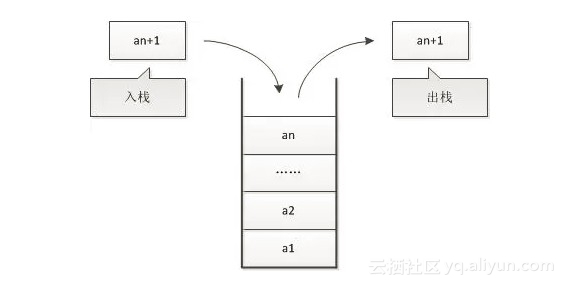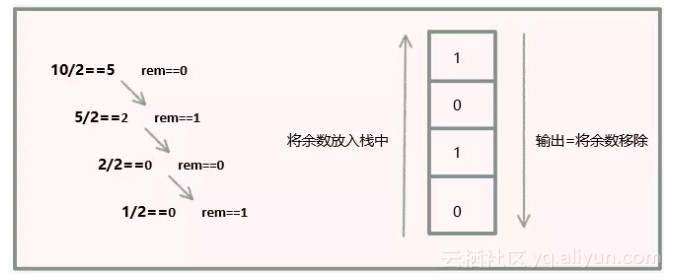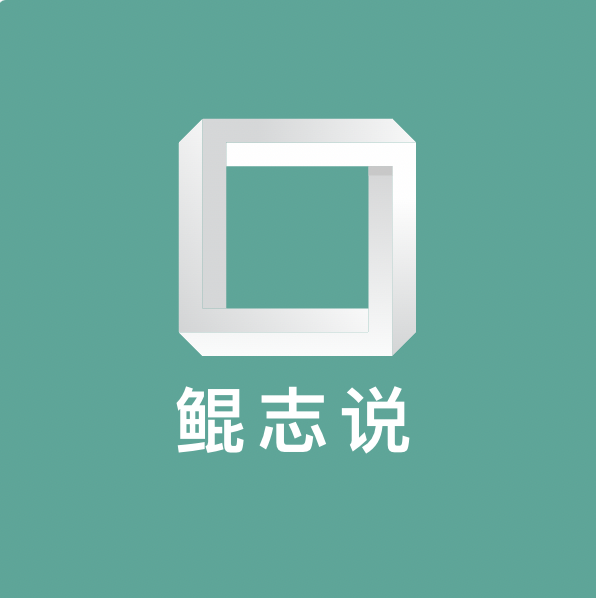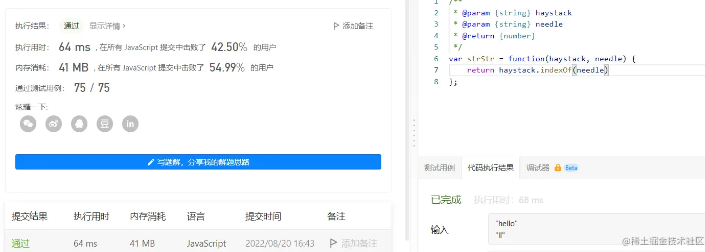+关注继续查看

# 什么是栈（Stack）• 栈是一种遵从后进先出（LIFO）原则的有序集合。
• 新添加的或待删除的元素都保存在栈的末尾，称为栈顶，另一端叫栈底。
• 在栈里，新元素都靠近栈顶，旧元素都接近栈底

# 手动实现一个栈

function Stack () { }

function Stack(){
var items = [];     //用来保存栈里的元素
}

push(element(s));   //添加新元素到栈顶
pop();              //移除栈顶的元素，同时返回被移除的元素
peek();             //返回栈顶的元素，不对栈做任何修改
isEmpty();          //如果栈里没有任何元素就返回true，否则false
clear();            //移除栈里的所有元素
size();             //返回栈里的元素个数,类似于数组的length属性


this.push = function (element) {
items.push(element);
}

this.pop = function () {
return items.pop();
}

this.peek = function () {
return items[items.length-1];
}

this.isEmpty = function () {
return items.length == 0;
}

this.size = function () {
return items.length;
}

this.clear = function () {
items = [];
}

this.print = function () {
console.log(items.toString());
}

# 栈的完整代码

function Stack(){

var items = [];     //用来保存栈里的元素

this.push = function (element) {
items.push(element);
}

this.pop = function () {
return items.pop();
}

this.peek = function () {
return items[items.length-1];
}

this.isEmpty = function () {
return items.length == 0;
}

this.size = function () {
return items.length;
}

this.clear = function () {
items = [];
}

this.print = function () {
console.log(items.toString());
}
}


# 使用Stack类

var stack = new Stack();
console.log(stack.isEmpty());         //控制台输出true

stack.push(5);
stack.push(8);

console.log(stack.peek());            //控制台输出8

stack.push(11);
console.log(stack.size());            //控制台输出3

stack.push(15);

stack.pop();
stack.pop();
console.log(stack.size());            //控制台输出2
stack.print();                        //控制台输出[5,8]

# 用栈来解决问题function divideBy2 (decNumber) {

var remStack = new Stack(),
rem,
binaryString = '';

while (decNumber>0) {  //{1}
rem = Math.floor(decNumber % 2);  //{2}
remStack.push(rem);  //{3}
decNumber = Math.floor(decNumber / 2);  //{4}
}

while (!remStack.isEmpty()) {  //{5}
binaryString += remStack.pop().toString();
}

return binaryString;
}


console.log(divideBy2(520));      //输出1000001000
console.log(divideBy2(10));       //输出1010
console.log(divideBy2(1000));     //输出1111101000


function baseConverter (decNumber, base) {

var remStack = new Stack(),
rem,
baseString = '';
digits = '0123456789ABCDEF';     //{6}

while (decNumber>0) {
rem = Math.floor(decNumber % base);
remStack.push(rem);  //{3}
decNumber = Math.floor(decNumber / base);
}

while (!remStack.isEmpty()) {
baseString += digits[remStack.pop()];  //{7}
}

return baseString;
}


console.log(baseConverter(1231,2));      //输出10011001111
console.log(baseConverter(1231,8));      //输出2317
console.log(baseConverter(1231,16));     //输出4CF


# 小结0 0JavaScript函数柯里化的实现原理，进来教你完成一个自己的自动实现柯里化方法
JavaScript函数柯里化的实现原理，进来教你完成一个自己的自动实现柯里化方法
0 0weex-自定义module，实现weex在iOS的本地化，js之间互相跳转，交互，传值（iOS接入weex的最佳方式）
weex-自定义module，实现weex在iOS的本地化，js之间互相跳转，交互，传值（iOS接入weex的最佳方式）
0 0js
0 0JS中实现或退出全屏
JS中实现或退出全屏
0 00 0【前端算法】JS实现数字千分位格式化
JS实现数字千分位格式化的几种思路，以及它们之间的性能比较
0 0【前端算法】用JS实现快速排序

0 0【前端算法】javaScript实现二分查找

0 00 0

JavaScript 自学手册文档教程313565

JavaScript入门与实战1896352

Javascript中的函数

Javascript异步编程

JS零基础入门教程（上册）